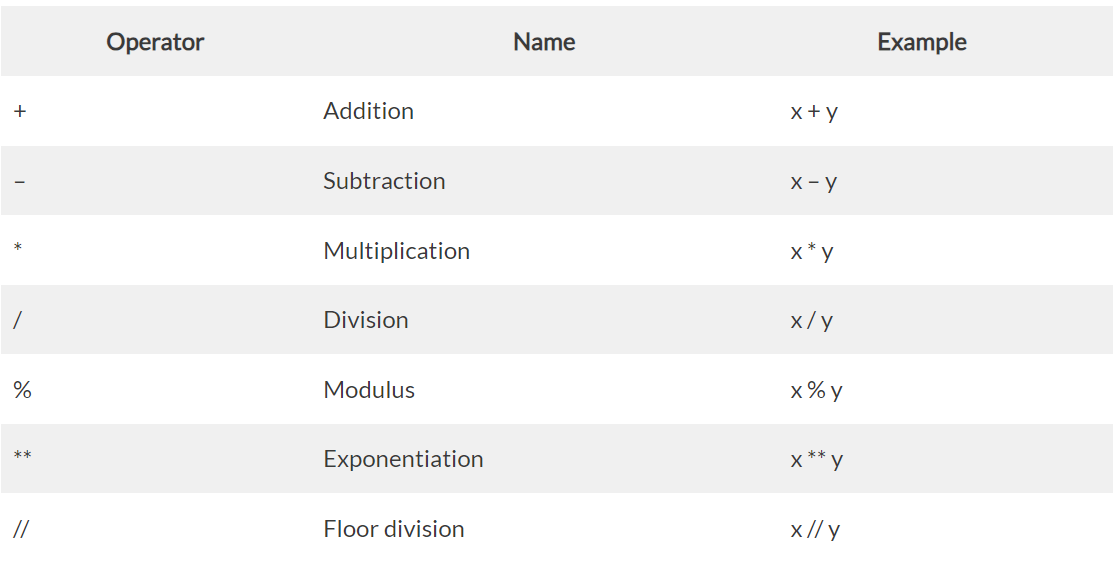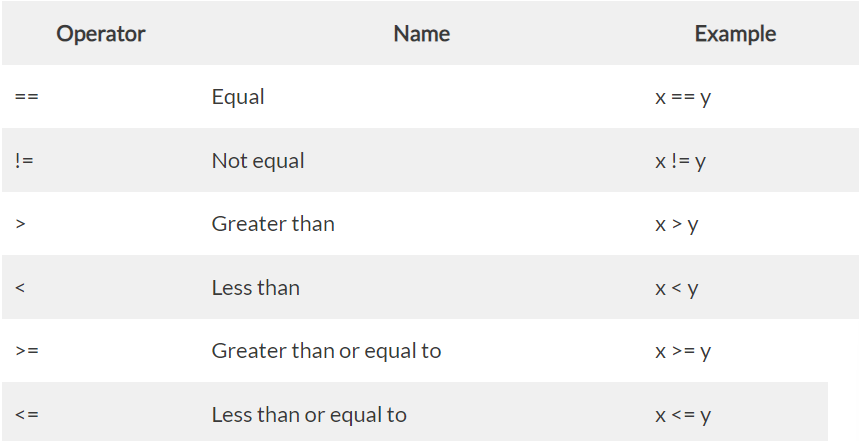Sat. Dec 2nd, 2023

# Operators – Arithmetic, Bitwise, comparison and Assignment operators, Operators Precedence and associativity

## Operators

Operators are used to perform operations on variables and values.

Python divides the operators in the following groups:

• Arithmetic operators
• Assignment operators
• Comparison operators
• Logical operators
• Identity operators
• Membership operators
• Bitwise operators

### Python Arithmetic Operators

Arithmetic operators are used with numeric values to perform common mathematical operations:Example:

Python Assignment Operators

Assignment operators are used to assign values to variables.

Python Comparison Operators

Comparison operators are used to compare two values:Example:

Python Logical Operators

Logical operators are used to combine conditional statements:

Example:

## Python Identity Operators

Identity operators are used to compare the objects, not if they are equal, but if they are actually the same object, with the same memory location:

Example:

Python Membership Operators

Membership operators are used to test if a sequence is presented in an object:

Example:

Python Bitwise Operators

Bitwise operators are used to compare (binary) numbers:

Operators Precedence

There can be more than one operator in an expression. To evaluate these types of expressions there is a rule of precedence in Python. It guides the order in which these operations are carried out. For example, multiplication has higher precedence than subtraction.

Python has well-defined rules for specifying the order in which the operators in an expression are evaluated when the expression has several operators. For example, multiplication and division have a higher precedence than addition and subtraction. Precedence rules can be overridden by explicit parentheses.

#### Precedence Order

When two operators share an operand, the operator with the higher precedence goes first. For example, since multiplication has a higher precedence than addition, a + b * c is treated as a + (b * c), and a * b + c is treated as (a * b) + c

### Precedence of Python Operators

The combination of values, variables, operators, and function calls is termed as an expression. The Python interpreter can evaluate a valid expression.

For example, multiplication has higher precedence than subtraction.

The operator precedence in Python is listed in the following table. It is in descending order (upper group has higher precedence than the lower ones).

Example for precedence of or & and:

Associativity in Python Operators

We can see in the above table that more than one operator exists in the same group. These operators have the same precedence.

When two operators have the same precedence, associativity helps to determine the order of operations.

Associativity is the order in which an expression is evaluated that has multiple operators of the same precedence. Almost all the operators have left-to-right associativity.

For example, multiplication and floor division have the same precedence. Hence, if both of them are present in an expression, the left one is evaluated first.

# Conditions(If else,if-elif-else)

## Conditional Statements

Python supports the usual logical conditions from mathematics:

Equals: a == b

Not Equals: a != b

Less than: a < b

Less than or equal to: a <= b

Greater than: a > b

Greater than or equal to: a >= b

### “If” condition

An “if statement” is written by using the if keyword.

### Indentation

Python relies on indentation (whitespace at the beginning of a line) to define scope in the code. Other programming languages often use curly-brackets for this purpose.

### Elif

The elif keyword is pythons way of saying “if the previous conditions were not true, then try this condition”.

### else

The else keyword catches anything which isn’t caught by the preceding conditions.

### Short hand if

If you have only one statement to execute, you can put it on the same line as the if statement.

### Short Hand If … Else

If you have only one statement to execute, one for if, and one for else, you can put it all on the same line.

### Nested if

You can have if statements inside if statements, this is called nested if statements.

# Loops(While ,for)

## Loops

### while loop

With the while loop we can execute a set of statements as long as a condition is true.

#### The else Statement

With the else statement we can run a block of code once when the condition no longer is true.

### for loop

A for loop is used for iterating over a sequence (that is either a list, a tuple, a dictionary, a set, or a string).

This is less like the for keyword in other programming languages, and works more like an iterator method as found in other object-orientated programming languages.

With the for loop we can execute a set of statements, once for each item in a list, tuple, set etc.

### Looping Through a String

Even strings are iterable objects, they contain a sequence of characters.

### The range() Function

To loop through a set of code a specified number of times, we can use the range() function,

The range() function returns a sequence of numbers, starting from 0 by default, and increments by 1 (by default), and ends at a specified number.

### Else in For Loop

The else keyword in a for loop specifies a block of code to be executed when the loop is finished.

### Nested Loops

A nested loop is a loop inside a loop.

The “inner loop” will be executed one time for each iteration of the “outer loop”:

# Break and Continue statements

### Break statement

The break statement terminates the loop containing it. Control of the program flows to the statement immediately after the body of the loop.

If the break statement is inside a nested loop the break statement terminates the innermost loop.

### Continue statement

The continue statement is used to skip the rest of the code inside a loop for the current iteration only. Loop doesn’t terminate but continues on with the next iteration.

### Pass statement

A pass statement is a null statement. The difference between a comment and a pass statement in Python is that while the interpreter ignores a comment entirely, pass is not ignored.

# Range functions

### Definition and Usage

The range() function returns a sequence of numbers, starting from 0 by default, and increments by 1 (by default), and stops before a specified number.

#### Example:

To create a sequence of numbers from 0 to 8, print each item in the sequence.

### Parameter Values

#### Example:

To create a sequence of numbers from 4 to 10, and print each item in sequence.

#### Example:

To create a sequence of numbers from 3 to 20 with an increment of 3 instead of 1.#### By Rajashekar

I’m (Rajashekar) a core Android developer with complimenting skills as a web developer from India. I cherish taking up complex problems and turning them into beautiful interfaces. My love for decrypting the logic and structure of coding keeps me pushing towards writing elegant and proficient code, whether it is Android, PHP, Flutter or any other platforms. You would find me involved in cuisines, reading, travelling during my leisure hours.